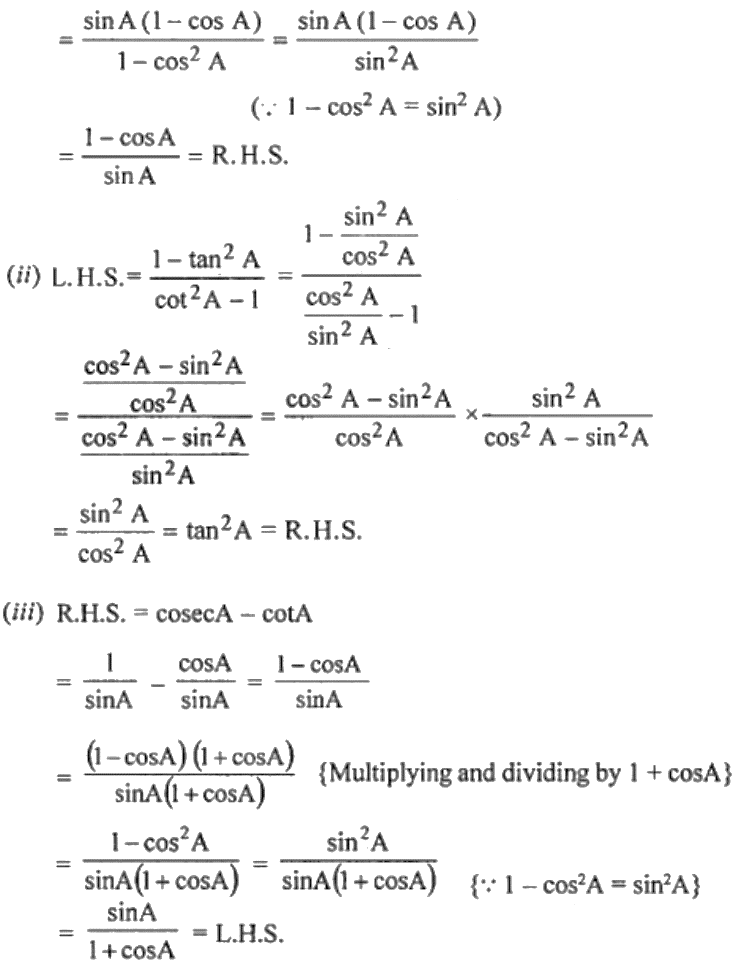Guru

# (i) sin A/ (1 + cos A) = (1 – cos A)/ sin A (ii) (1 – tan2 A)/ (cot2 A – 1) = tan2 A (iii) sin A/ (1 + cos A) = cosec A – cot A

• 0

This is an important question taken from the Book ML Aggarwal, Avichal publication, class 10th and, chapter 18

We have to proof that

(i) sin A/ (1 + cos A) = (1 – cos A)/ sin A

(ii) (1 – tan2 A)/ (cot2 A – 1) = tan2 A

(iii) sin A/ (1 + cos A) = cosec A – cot A

Class10th, chapter 18, ques no 15, trigonometric identities

Share